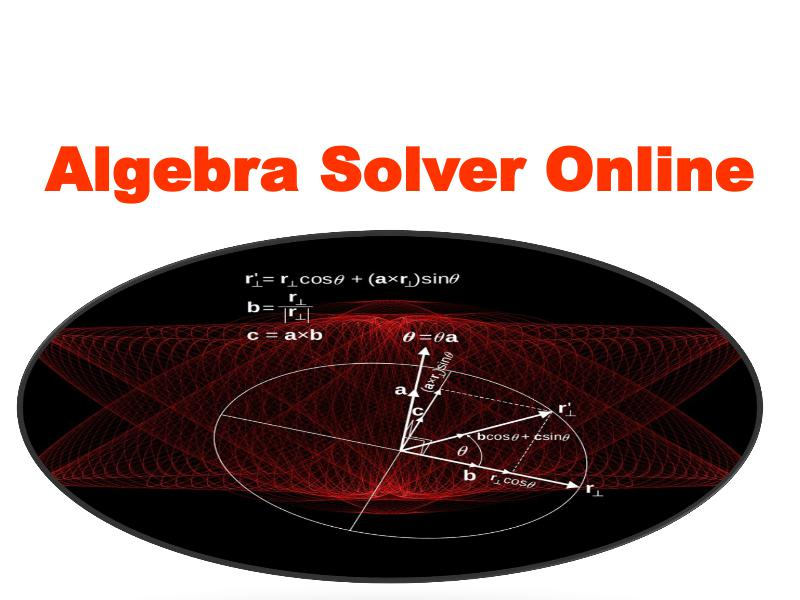点击阅读
• 评论

# Algebra Solver Online1

Manipulation of Algebraic equations has for long remained one of the most challenging areas in Mathematics. Handling Algebra implies dealing with a combination of numbers, letters, mathematical symbol 更多
Manipulation of Algebraic equations has for long remained one of the most challenging areas in Mathematics. Handling Algebra implies dealing with a combination of numbers, letters and other mathematical symbols that are not necessarily whole numbers. 更多